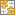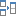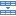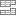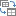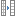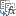# Excel ISBLANK function to check if cell is empty or not

The tutorial shows how to use ISBLANK and other functions to identify blank cells in Excel and take different actions depending on whether a cell is empty or not.

There are many situations when you need to check if a cell is empty or not. For instance, if cell is blank, then you might want to sum, count, copy a value from another cell, or do nothing. In these scenarios, ISBLANK is the right function to use, sometimes alone, but most often in combination with other Excel functions.

## Excel ISBLANK function

The ISBLANK function in Excel checks whether a cell is blank or not. Like other IS functions, it always returns a Boolean value as the result: TRUE if a cell is empty and FALSE if a cell is not empty.

The syntax of ISBLANK assumes just one argument:

ISBLANK (value)

Where value is a reference to the cell you want to test.

For example, to find out if cell A2 is empty, use this formula:

`=ISBLANK(A2)`

To check if A2 is not empty, use ISBLANK together with the NOT function, which returns the reversed logical value, i.e. TRUE for non-blanks and FALSE for blanks.

`=NOT(ISBLANK(A2))`

Copy the formulas down to a few more cells and you will get this result: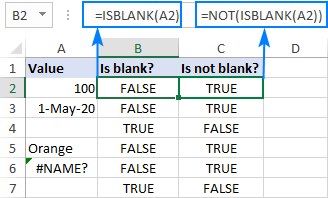### ISBLANK in Excel - things to remember

The main point you should keep in mind is that the Excel ISBLANK function identifies truly empty cells, i.e. cells that contain absolutely nothing: no spaces, no tabs, no carriage returns, nothing that only appears blank in a view.

For a cell that looks blank, but in fact is not, an ISBLANK formula returns FALSE. This behavior occurs if a cell contains any of the following:

• Formula that returns an empty string like IF(A1<>"", A1, "").
• Zero-length string imported from an external database or resulted from a copy/paste operation.
• Spaces, apostrophes, non-breaking spaces (&nbsp;), linefeed or other non-printing characters.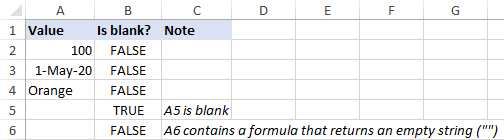## How to use ISBLANK in Excel

To gain more understanding of what the ISBLANK function is capable of, let's take a look at some practical examples.

### Excel formula: if cell is blank then

Since Microsoft Excel does not have a built-in IFBLANK kind of function, you need to use IF and ISBLANK together to test a cell and perform an action if the cell is empty.

Here's the generic version:

IF(ISBLANK(cell), "if blank", "if not blank")

To see it in action, let's check if a cell in column B (delivery date) has any value in it. If the cell is blank, then output "Open"; if the cell is not blank, then output "Completed".

`=IF(ISBLANK(B2), "Open", "Completed")`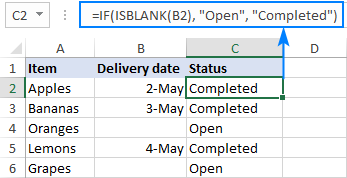Please remember that the ISBLANK function only determines absolutely blank cells. If a cell contains something invisible to the human eye such as a zero-length string, ISBLANK would return FALSE. To illustrate this, please have a look at the screenshot below. The dates in column B are pulled from another sheet with this formula:

`=IF(Sheet3!B2<>"",Sheet3!B2,"")`

As the result, B4 and B6 contain empty strings (""). For these cells, our IF ISBLANK formula yields "Completed" because in terms of ISBLANK the cells are not empty.

If your classification of "blanks" includes cells containing a formula that results in an empty string, then use ="" for the logical test:

`=IF(B2="", "Open", "Completed")`

The screenshot below shows the difference: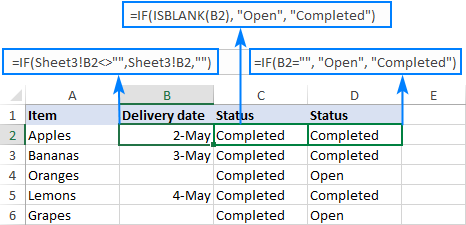### Excel formula: if cell is not blank then

If you've closely followed the previous example and understood the formula's logic, you should have no difficulties with modifying it for a specific case when an action shall only be taken when the cell is not empty.

Based on your definition of "blanks", choose one of the following approaches.

To identify only truly non-blank cells, reverse the logical value returned by ISBLANK by wrapping it into NOT:

IF(NOT(ISBLANK(cell)), "if not blank", "")

Or use the already familiar IF ISBLANK formula (please notice that compared to the previous one, the value_if_true and value_if_false values are swapped):

IF(ISBLANK(cell), "", if not blank")

To teat zero-length strings as blanks, use <>"" for the logical test of IF:

IF(cell <>"", "if not blank", "")

For our sample table, any of the below formulas will work a treat. They all will return "Completed" in column C if a cell in column B is not empty:

`=IF(NOT(ISBLANK(B2)), "Completed", "")`

`=IF(ISBLANK(B2), "", "Completed")`

`=IF(B2<>"", "Completed", "")`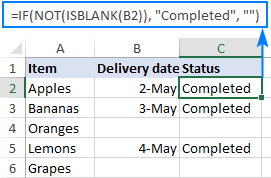### If cell is blank, then leave blank

In certain scenarios, you may need a formula of this kind: If cell is blank do nothing, otherwise take some action. In fact, it's nothing else but a variation of the generic IF ISBLANK formula discussed above, in which you supply an empty string ("") for the value_if_true argument and the desired value/formula/expression for value_if_false.

For absolutely blank cells:

IF(ISBLANK(cell), "", if not blank")

To regard empty strings as blanks:

IF(cell="", "", if not blank")

In the table below, suppose you want to do the following:

• If column B is empty, leave column C empty.
• If column B contains a sales number, calculate the 10% commission.

To have it done, we multiply the amount in B2 by percentage and put the expression in the third argument of IF:

`=IF(ISBLANK(B2), "", B2*10%)`

Or

`=IF(B2="", "", B2*10%)`

After copying the formula through column C, the result looks as follows: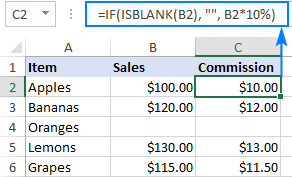### If any cell in range is blank, then do something

In Microsoft Excel, there are a few different ways to check a range for empty cells. We will be using an IF statement to output one value if there is at least one empty cell in the range and another value if there are no empty cells at all. In the logical test, we calculate the total number of empty cells in the range, and then check if the count is greater than zero. This can be done with either COUNTBLANK or COUNTIF function:

COUNTBLANK(range)>0
COUNTIF(range,"")>0

Or a little bit more complex SUMPRODUCT formula:

SUMPRODUCT(--(range=""))>0

For example, to assign the "Open" status to any project that has one or more blanks in columns B through D, you can use any of the below formulas:

`=IF(COUNTBLANK(B2:D2)>0,"Open", "")`

`=IF(COUNTIF(B2:D2,"")>0, "Open", "")`

`=IF(SUMPRODUCT(--(B2:D2=""))>0, "Open", "")`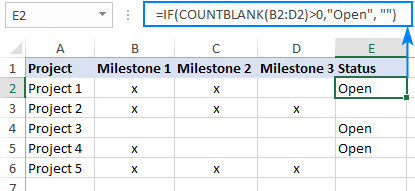Note. All these formulas treat empty strings as blanks.

### If all cells in range are blank, then do something

To check if all cells in the range are empty, we will be using the same approach as in the above example. The difference is in the logical test of IF. This time, we count cells that are not empty. If the result is greater than zero (i.e. the logical test evaluates to TRUE), we know that not every cell in the range is blank. If the logical test is FALSE, that means all cells in the range are blank. So, we supply the desired value/expression/formula in the 3rd argument of IF (value_if_false).

In this example, we will return "Not Started" for projects that have blanks for all the milestones in columns B through D.

The easiest way to count non-empty cells in Excel is by using the COUNTA function:

`=IF(COUNTA(B2:D2)>0, "", "Not Started")`

Another way is COUNTIF for non-blanks ("<>" as the criteria):

`=IF(COUNTIF(B2:D2,"<>")>0, "", "Not Started")`

Or the SUMPRODUCT function with the same logic:

`=IF(SUMPRODUCT(--(B2:D2<>""))>0, "", "Not Started")`

ISBLANK can also be used, but only as an array formula, which should be completed by pressing Ctrl + Shift + Enter, and in combination with the AND function. AND is needed for the logical test to evaluate to TRUE only when the result of ISBLANK for each cell is TRUE.

`=IF(AND(ISBLANK(B2:D2)), "Not Started", "")`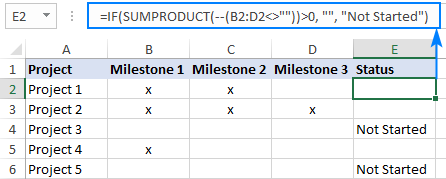Note. When choosing a formula for your worksheet, an important thing to consider is your understanding of "blanks". The formulas based on ISBLANK, COUNTA and COUNTIF with "<>" as the criteria look for absolutely empty cells. SUMPRODUCT also regards empty strings as blanks.

### Excel formula: if cell is not blank, then sum

To sum certain cells when other cells are not blank, use the SUMIF function, which is especially designed for conditional sum.

In the table below, supposing you wish to find the total amount for the items that are already delivered and those that are not yet delivered.

#### If not blank then sum

To get the total of delivered items, check if the Delivery date in column B is not blank and if it isn't, then sum the value in column C:

`=SUMIF(B2:B6, "<>", C2:C6)`

#### If blank then sum

To get the total of undelivered items, sum if the Delivery date in column B is blank:

`=SUMIF(B2:B6, "", C2:C6)`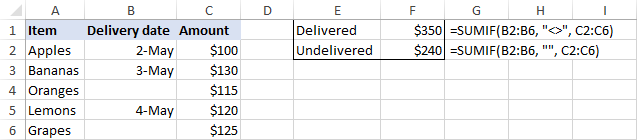### Sum if all cells in range are not blank

To sum cells or perform some other calculation only when all cells in a given range are not blank, you can again use the IF function with the appropriate logical test.

For example, COUNTBLANK can bring us the total number of blanks in the range B2:B6. If the count is zero, we run the SUM formula; otherwise do nothing:

`=IF(COUNTBLANK(B2:B6)=0, SUM(B2:B6), "")`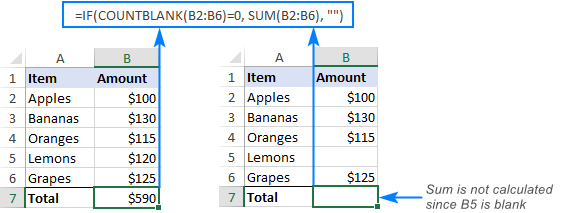The same result can be achieved with an array IF ISBLANK SUM formula (please remember to press Ctrl + Shift + Enter to complete it correctly):

`=IF(OR(ISBLANK(B2:B6)), "", SUM(B2:B6))`

In this case, we use ISBLANK in combination with the OR function, so the logical test is TRUE if there is at least one blank cell in the range. Consequently, the SUM function goes to the value_if_false argument.

### Excel formula: count if cell is not blank

As you probably know, Excel has a special function to count non-empty cells, the COUNTA function. Please be aware that the function counts cells containing any type of data, including the logical values of TRUE and FALSE, error, spaces, empty strings, etc.

For example, to count non-blank cells in the range B2:B6, this is the formula to use:

`=COUNTA(B2:B6)`

The same result can be achieved by using COUNTIF with the non-blank criteria ("<>"):

`=COUNTIF(B2:B6,"<>")`

To count blank cells, use the COUNTBLANK function:

`=COUNTBLANK(B2:B6)`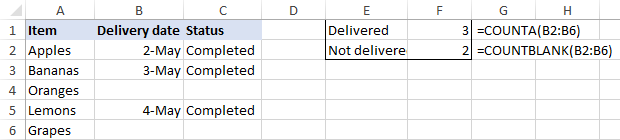## Excel ISBLANK not working

As already mentioned, ISBLANK in Excel returns TRUE only for really empty cells that contain absolutely nothing. For seemingly blank cells containing formulas that produce empty strings, spaces, apostrophes, non-printing characters, and the like, ISBLANK returns FALSE.

In a situation, when you want to treat visually empty cells as blanks, consider the following workarounds.

### Treat zero-length strings as blanks

To consider cells with zero-length strings as blanks, in the logical test of IF, put either an empty string ("") or the LEN function equal to zero.

`=IF(A2="", "blank", "not blank")`

Or

`=IF(LEN(A2)=0, "blank", "not blank")`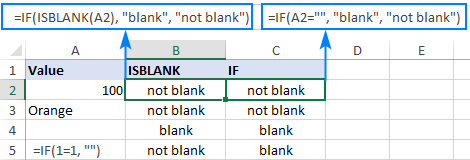### Remove or ignore extra spaces

In case the ISBLANK function is malfunctioning because of blank spaces, the most obvious solution is to get rid of them. The following tutorial explains how to quickly remove leading, trailing and multiple in-between spaces, except for a single space character between words: How to remove extra spaces in Excel.

If for some reason removing excess spaces does not work for you, you can force Excel to ignore them.

To regard cells containing only space characters as empty, include LEN(TRIM(cell))=0 in the logical test of IF as an additional condition:

`=IF(OR(A2="", LEN(TRIM(A2))=0), "blank", "not blank")`

To ignore a specific non-printing character, find its code and supply it to the CHAR function.

For example, to identify cells containing empty strings and nonbreaking spaces (&nbsp;) as blanks, use the following formula, where 160 is the character code for a nonbreaking space:

`=IF(OR(A2="", A2=CHAR(160)), "blank", "not blank")`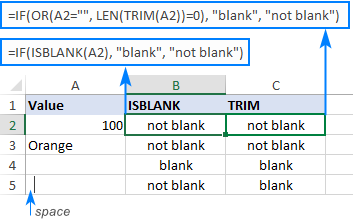That's how to use the ISBLANK function to identify blank cells in Excel. I thank you for reading and hope to see you on our blog next week!

Excel ISBLANK formula examples

### 10 responses to "Excel ISBLANK function to check if cell is empty or not"

1.ahmed fatthi says:

thank u very much

2.Maryam Maishanu says:

Your presentations are superb. I wrote a similar If not blank then sum formula. However it didn't work and I knew the problem was with the logical test. I got to your page and saw that it should simply be written as while I wrote mine as "" to test for empty. I have made the corrections and it worked! However I am a little confused as to how the formula could work without testing for any specific condition. I will truly appreciate your explanations. Thanks

3.Paula Moore says:

I am trying to make a conditional formula that if cell A1 is blank, then I need cell B1 to be blank, but if cell A1 has a date that is today or older in the past, the B1 cell is to be highlighted and have a certain text color, but if the date in cell A1 is a future date (tomorrow and forward), I need cell B1 to be a different color and font. I am basically using it to alert me to things that are due to be worked because time is up. I want it to stick out that I need to work something because time is up today.

Paula

4.Earl says:

I would like to calculate a due date of 10 working days ONLY if the first column is not blank.
Cell H132 is my first calculated date (given to employee). Column J132 would be the target date of 10 working days after only if H132 is blank.

So, given that, =WORKDAY(H132,10) is fine. How do I incorporate to not count if H132 is blank?

I have tried both ISBLANK

•Earl says:

I tried this as well:
=if(or(isblank(H199),"",workday(H199,10,holidays!A1:a11)))

•Earl says:

=IF(ISBLANK(H67),"",WORKDAY(H67,10,Holidays!A1:A11))

5.Earl says:

I would like to calculate a due date of 10 working days ONLY if the first column is not blank.
Cell H132 is my first calculated date (given to employee). Column J132 would be the target date of 10 working days after only if H132 is blank.

So, given that, =WORKDAY(H132,10) is fine. How do I incorporate to not count if H132 is blank?

Previously, I was using "IF(H132="","",H132+10) which works fine. How do I incorporate the workday function? Thank you!

•Alexander Trifuntov (Ablebits.com Team) says:

Hello!

=IFERROR(IF(ISBLANK(H132),"",WORKDAY(H132,10)),"")

6.Earl says: